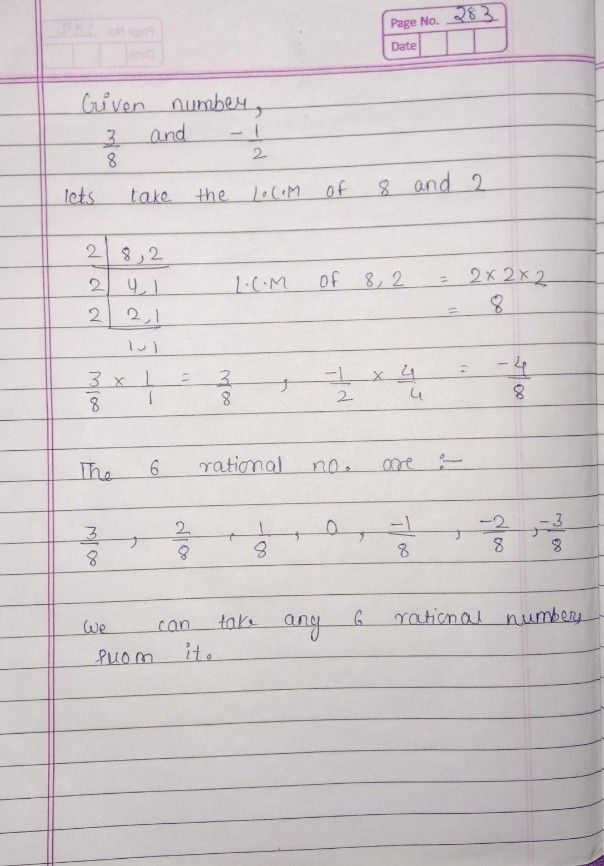Symbol
Problem$1\right)$ accurately construct mathematical models to represent $xea|-lit$ situations using functions; $2\right)$ apply the concepts of inverse functions, exponential functions, and $1$ logarithmic functions to formulate and solve $1$ $ca|-litc$ problems with precision and accuracy;
10th-13th grade
Other
Search count: 106
Question content
Please help me make an example and solve pleaseee
SolutionQanda teacher - bhargavi